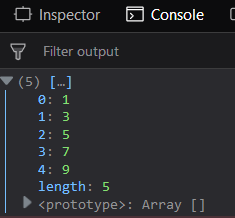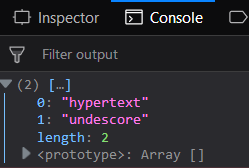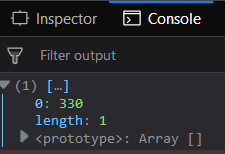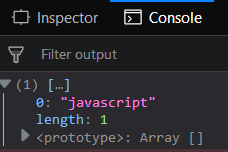# Underscore.js | _.filter() with Examples

The Underscore.js is a JavaScript library that provides a lot of useful functions like the map, filter, invoke etc even without using any built-in objects.

The _.filter() is used to check which elements in the passed array satisfy the condition. It will form a new array of all those elements which satisfy the condition passed from the array. It is mostly used when need to find certain elements from a large array.

Syntax:

`_.filter( list, predicate, [context] )`

Parameters: This function accept three parameters as mentioned above and described below:

• list: This parameter is used to hold the list of items.
• predicate: This parameter is used to hold the truth condition.
• context: The text content which need to be display. It is optional parameter.

Return value: It returns an array consisting of elements which satisfy the condition.

Passing a list of numbers to _.filter() function: The _.filter() function takes the element from the list one by one and checks the specified operations on the code. Like here the operation is to find the elements of the list is even or not. Only the odd elements will be added to the resultant array.

Example:

 ` ` `<``html``> ` `    ``<``head``> ` `        ``<``script` `type``=``"text/javascript"` `src``= ` `        ``"https://cdnjs.cloudflare.com/ajax/libs/underscore.js/1.9.1/underscore-min.js"` `> ` `        `` ` `    `` ` `    ``<``body``> ` `        ``<``script` `type``=``"text/javascript"``> ` `            ``var oddNo = _.filter([1, 2, 3, 4, 5, 6, 7, 8, 9, 10],  ` `                ``function(num){  ` `                    ``return num % 2 != 0; ` `                ``}); ` `            ``console.log(oddNo); ` `        `` ` `    `` ` `                     `

Output:Passing a list of words to _.filter() function: The _.filter() function takes the element word from the list one by one and checks the specified operations on the code. Like here the operation is to find the elements of the list which have a length of 9. Only those words will be added to the resultant array whose length is equal to 9.

Example:

 ` ` `<``html``> ` `    ``<``head``> ` `        ``<``script` `type``=``"text/javascript"` `src``= ` `        ``"https://cdnjs.cloudflare.com/ajax/libs/underscore.js/1.9.1/underscore-min.js"` `> ` `        `` ` `    `` ` `    ``<``body``> ` `        ``<``script` `type``=``"text/javascript"``> ` `            ``var words = ['javascript', 'java', 'unix', 'hypertext', 'undescore', 'CSS']; ` `            ``const result = words.filter(word => word.length == 9); ` `            ``console.log(result); ` `        `` ` `    `` ` `                     `

Output:Passing a separate function to the _.filter(): Pass a user defined function to the _.filter() function. First declare the function like here the function name is ‘largest()’ which returns the element if it is greater than or equal to 100. This function can do any comparison as declared by the user. Then, in the _.filter pass this function. At the end console.log) the resultant array.

Example:

 ` ` `<``html``> ` `    ``<``head``> ` `        ``<``script` `type``=``"text/javascript"` `src``=  ` `        ``"https://cdnjs.cloudflare.com/ajax/libs/underscore.js/1.9.1/underscore-min.js"` `> ` `        `` ` `    ``  ` `    ``<``body``> ` `        ``<``script` `type``=``"text/javascript"``> ` `            ``function largest(v) { ` `                ``return v >= 100; ` `            ``} ` `            ``var res = [1, 4, 12, 15, 8, 330, 54].filter(largest); ` `            ``console.log(res); ` `        `` ` `    ``  ` `                     `

Output:Using other functions with the _.reduce() function: Use toLowerCase() and indexOf() functions in the _.filter() function. First find the index of each element and then check whether it is greater than -1. Since console.log() is used at the end therefore the output will only be seen for the last element of the passed array.

Example:

 ` ` `<``html``>  ` `    ``<``head``> ` `        ``<``script` `type``=``"text/javascript"` `src``= ` `        ``"https://cdnjs.cloudflare.com/ajax/libs/underscore.js/1.9.1/underscore-min.js"` `> ` `        `` ` `    ``  ` `    ``<``body``> ` `        ``<``script` `type``=``"text/javascript"``> ` `            ``const lang = ['hypertext', 'markup', 'language', 'cascading', ` `                                        ``'style', 'sheet', 'javascript']; ` `            ``const func = (query) =>  ` `            ``{ ` `                ``return lang.filter((el) => ` `                    ``el.toLowerCase().indexOf(query.toLowerCase()) > -1 ` `                ``); ` `            ``} ` `            ``console.log(func('pt'));  ` `        `` ` `    ``  ` `                     `

Output:Note: These commands will not work in Google console or in Firefox as for these additional files need to be added which they didn’t have added. So, add the given links to your HTML file and then run them.

 `<``script` `type``=``"text/javascript"` `src` `=  ` `"https://cdnjs.cloudflare.com/ajax/libs/underscore.js/1.9.1/underscore-min.js"``>  ` `  `My Personal Notes arrow_drop_upCheck out this Author's contributed articles.

If you like GeeksforGeeks and would like to contribute, you can also write an article using contribute.geeksforgeeks.org or mail your article to contribute@geeksforgeeks.org. See your article appearing on the GeeksforGeeks main page and help other Geeks.

Please Improve this article if you find anything incorrect by clicking on the "Improve Article" button below.

Improved By : shubham_singh

Article Tags :

Be the First to upvote.

Please write to us at contribute@geeksforgeeks.org to report any issue with the above content.